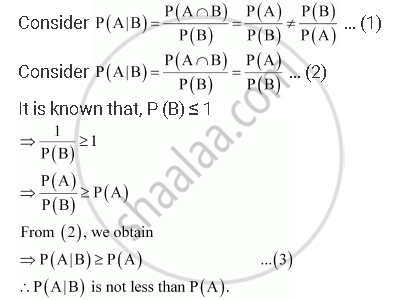Share

# If a and B Are Two Events Such that a ⊂ B and P (B) ≠ 0, Then Which of the Following is Correct? - CBSE (Science) Class 12 - Mathematics

#### Question

If A and B are two events such that A ⊂ B and P (B) ≠ 0, then which of the following is correct?

A. P(A |B) = (P(B))/(P(A))

B. P(A|B) < P(A)

C. P(A|B) >= P(A)

D. None of these

#### Solution

If A ⊂ B, then A ∩ B = A

⇒ P (A ∩ B) = P (A)

Also, P (A) < P (B)Thus, from (3), it can be concluded that the relation given in alternative C is correct.

Is there an error in this question or solution?

#### APPEARS IN

Solution If a and B Are Two Events Such that a ⊂ B and P (B) ≠ 0, Then Which of the Following is Correct? Concept: Baye'S Theorem.
S